The Gecko -- is the most intriguing of Dragons. Colourful but bereft of eyelids, lacking claws, yet so provocative. Aristotle insisted that the gecko can run up and down a tree in any way, even with the head downwards. Its physics -- how the gecko climbs walls and even across ceilings -- is beyond Aristotle and Newton -- and involves quantum physics. [ Not that such advanced physics is required to dissect and analyse my geckos.] When challenged by a gecko many try to bar this creature -- but the more creative task is to "tame" [adjust] the gecko so as to transform it to something more familiar. The genus was properly first described in problem-solving terms by Imre Lakatos, hence the alternate nomenclaure Lakatosian Monster. Dragons are formidable problems, challenging, and yet solvable using little or even no algebra or calculus. But their solutions requires one to use or develop genuine physical insight, beyond that acquired in childhood. For more on dragons click hereEastern Water Dragon, in Sydney Harbour bush.

On Lakatosian Monsters

In his classic work, Proofs and Refutationas, Imre Lakatos explored how mathematics develops in practice. He choose as his model for study the evolution of proofs published in the mathematics literature, both in papers and texts, of the proof of Euler's Formula, that relates
• E = the number of edges
• V = the number of vertices
• F = the number of faces
for three dimensional polyhedra according to the equation
V + F - E = 2
This formula is very easily checked for the five platonic solids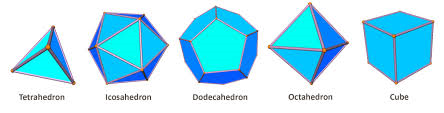V=4 V=12 V=20 V=5 V=8
F=4 F=20 F=12 F=6 F=6
E=6 E=30 E=30 E=9 E=12

and it works perfectly for familiar convex polyhedra.
Latatos showed how with the discovery of various counter-examples -- polyhedra for which Euler's Formula does not hold -- the published "proofs" of Euler's Formula, evolved over time.
These counter-examples were termed by Lakatos monsters.
Lakatos delineated two major procedures for dealing with monsters:
• monster-barring
as well as what he termed exception barring.

Most striking of the monsters that Lakatos discussed wereThe Great Dodecahedron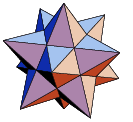Small Stellated Dodecahedron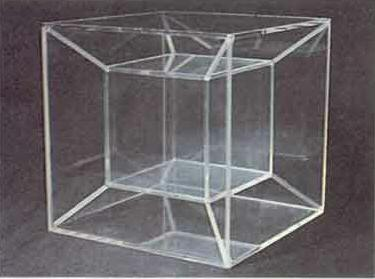The Nested Cube [ Wireframe ] V=12 V=12 V=16 F=12 F=12 F=20 E=30 E=30 E=32 V+F-E=-6 V+F-E=-6 V+F-E=4

[ Note the use of specific colours for each visible face of the monstrous dodecahedrons ]
The original articles and the 1976 book by Lakatos, Proofs and Refutations are not freely available online. However this writer recommends for academic readers two online publications: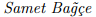andAn Examination of Counterexamples in Proofs and Refutations
Jesse Lambe Formal Proofs and Refutations, PhD thesis, Stanford, 2009
Now it must be said that while Lakatos was solely concerned with the historical development of proofs in pure mathematics. here his terminology has been applied by me to the description of problem solutions in qualitative physics.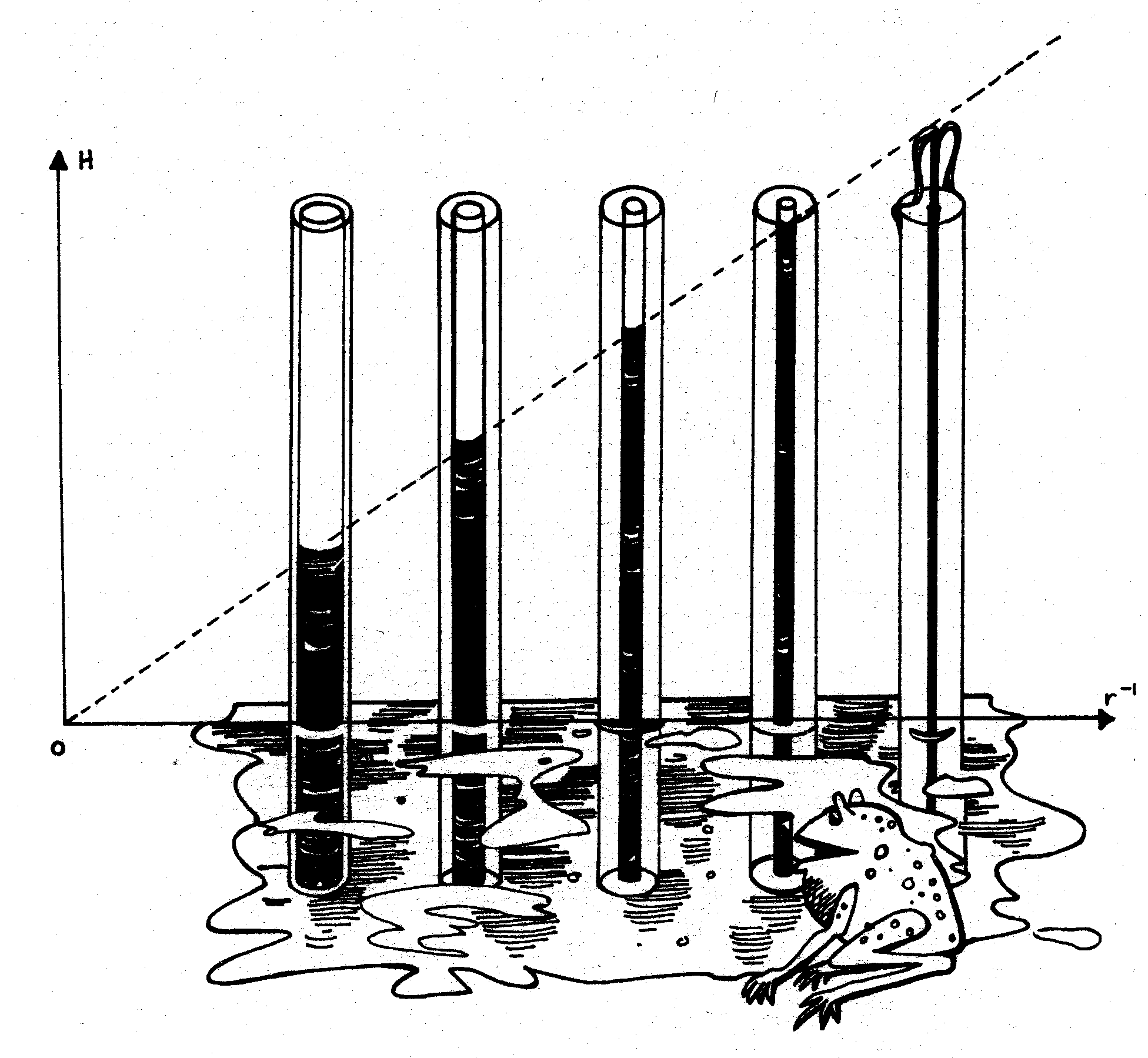Elementary physics solution: Water in a vertical capillary tube immersed in water will rise an amount     H = K/r Derivation as per many texts For surface tension T, and angle of contact θ a column of water (or like fluid) will be rise by amount H till the gravitational force on the column (above surroundings) is matched by the total surface tension, so that     2πrTsin(θ) = π r2 ρg H where ρ is the fluid density, and g is the force of gravity. Hence, introducing a constant K, the rise in the tube is computed as     H = K/r

In presenting this graphic Inducia Capillaria to a wide range of subjects I have added verbal reparté such as:
One sees that the narrower the tube -- the higher the water roses. Does that mean that for a thin enough tube water would spray out of the top of the tube? Or would water just dribble over the top of the tube?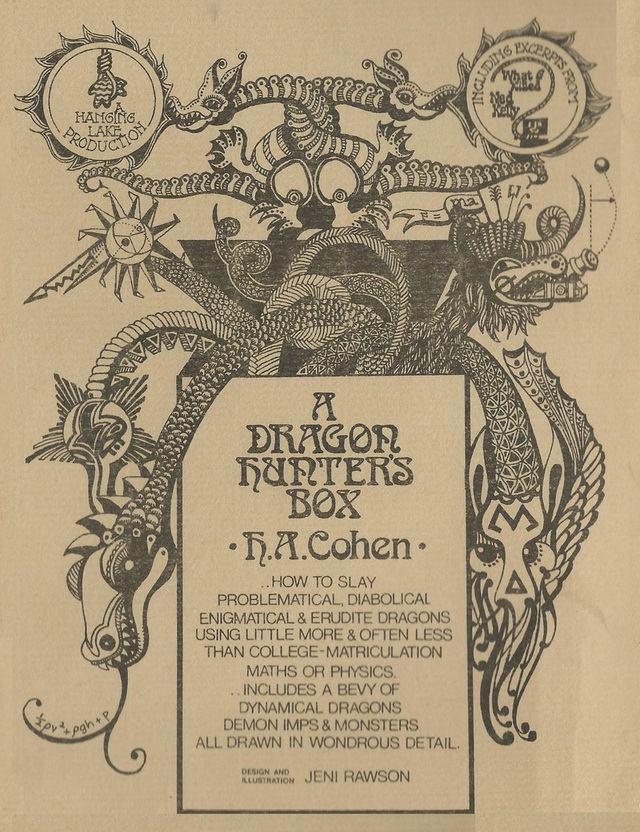1974 was the Year for Dragons and Monsters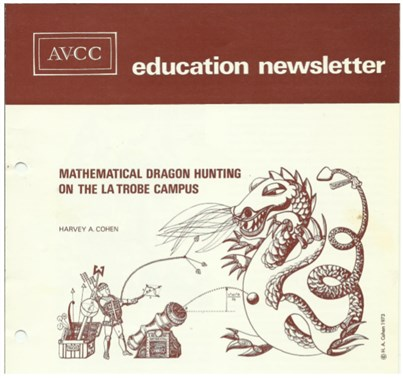Australian Vice-Chancellor"s Committee (AVCC) EducationalNewsletter, 3/73, January 1974 Downloadable.

Summary
I apply Lakatos's terminology to qualitative physics to specify the 'standard' methods of dealing with monsters are
• monster-barring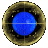The Art of Snaring DragonsInducia Capillaria IIDragon Home Page